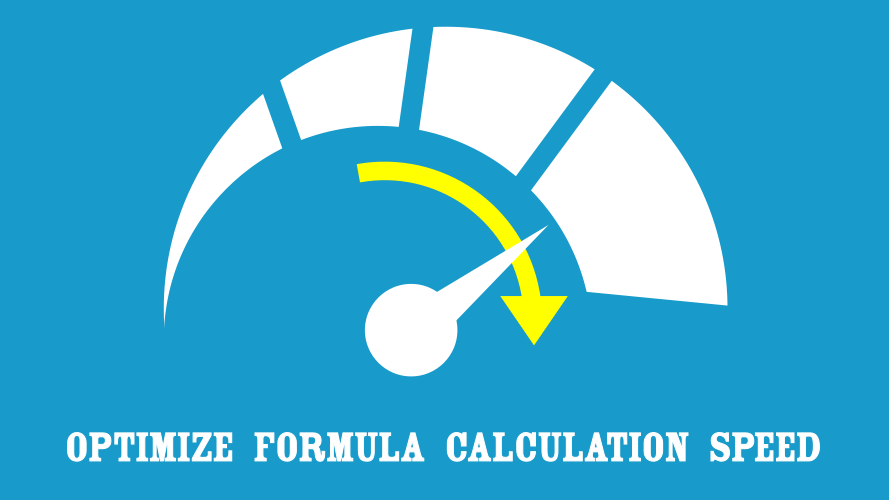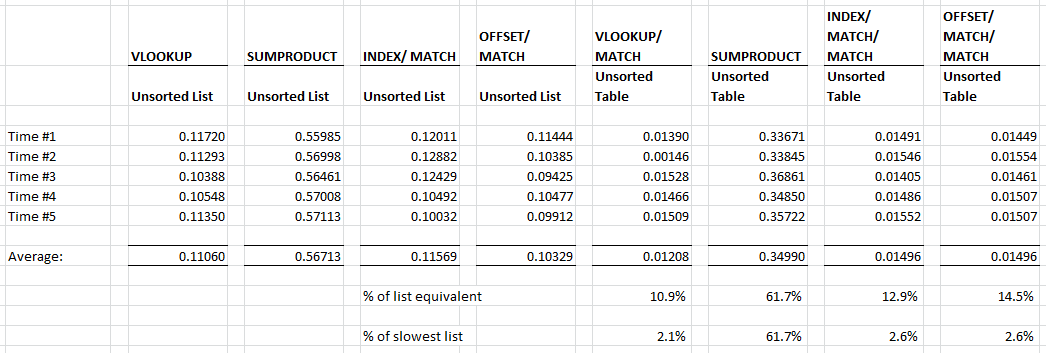# Optimize Formula Calculation Speed – Part 6: Table or list?## Part 6: Table or list?

In the previous part, we looked at the impact of having sorted or unsorted source data on the speed of various lookup functions. We discovered there is a significant time benefit on VLOOKUP, INDEX/MATCH and OFFSET/MATCH where the searched records appear earlier in the source data.

In this part, we are going to look at the speed difference which can be obtained by changing our source data from a list into a table.

I am a great believer that data should be presented in a manner which is still readable. So that, if we need to troubleshoot our formula for any reason it will be much easier to find the issue.  Whilst we could have used any of the dimensions for the columns in our table, the most sensible option to me seemed to be year and period.

## Setting up the test

For the list, we used the same 42,941 Data lines as used for Part 4 Lookup formulas and Part 5 Sorting source data.

The table we will use is based on the same information as the list, but with the Year and Period criteria in the columns and the remaining criteria to the left. This reduced the number of data rows to 1,620, but we now have 36 columns, rather than a single column.

The formulas we will be using are:

 List Table equivalent VLOOKUP VLOOKUP/MATCH SUMPRODUCT SUMPRODUCT INDEX/MATCH INDEX/MATCH/MATCH OFFSET/MATCH OFFSET/MATCH/MATCH

The formulas we used in the previous parts of SUMIF and SUM (Array) won’t work on a table layout, so I have not included these in the test.

All the data and workings from the tests can be downloaded here.

## The results

The results of the test are below:Of the methods using the table as a source VLOOKUP/MATCH was only slightly faster than INDEX/MATCH/MATCH and OFFSET/MATCH/MATCH. As expected, based on the previous parts, SUMPRODUCT was significantly slower.

When comparing the list and table data sources, the calculations based on the table were always faster than those based on the list . SUMPRODUCT calculated in approximately 60% of the time, but the real winners were the VLOOKUP/MATCH, INDEX/MATCH/MATCH and OFFSET/MATCH/MATCH formulas which calculated in 10%-15% of the time (that’s a significant time saving!!).

These speed gains are because Excel actually has significantly less records to sort through when performing a lookup on a table. The list has 42,941  lines, but the table only as 1,620 lines and 36 columns.  So that’s 1,656 items to sort through in total, which which is significantly smaller.

If we are on the hunt for speed improvements, then we might take a different decision about which columns to use. To optimize formula calculation speed the goal is to minimize the total rows plus total columns.  There is no set rule of this, it would depend on the data in each circumstance.

Also, notice that if we were using SUMPRODUCT on a list and changed to a VLOOKUP/MATCH formula on a table we could achieve significant speed benefits.   We could obtain the same result in 2.1% of the time (that’s approx. 50 times faster!!!).

## Conclusion

Having the source data as a table, rather than a list can significantly speed up the lookup formula. Whilst SUMPRODUCT maybe the Swiss Army Knife of lookup formulas (it can work on a list or on a table), it is consistently slower in both circumstances.

## Next time

So far we have lived in a world where we have looked up data based on 1 or 2 criteria.  But our source data actually has 6 criteria.  Next time we will look at formulas which can work with multiple criteria. We will see how they perform against each other.